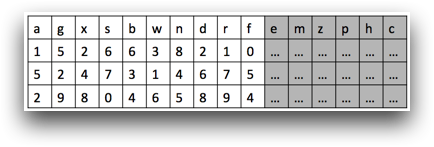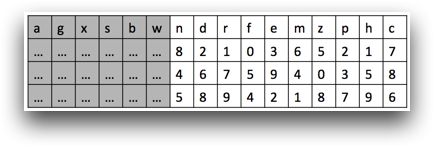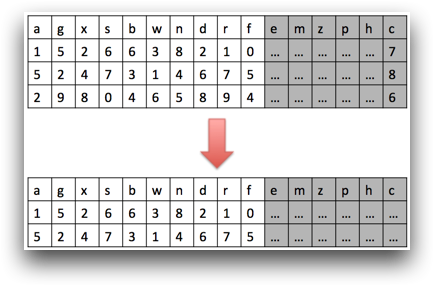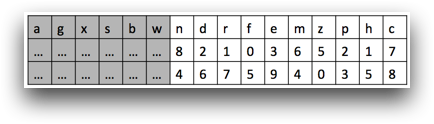Lazy evaluation

Certain operations in 1010data are not evaluated until the data from those operations are needed by another part of the query.

Key concepts

• <willbe> and <link> operations are lazily evaluated in 1010data.
• Results of <willbe> operations are not cached throughout a query but are cached within a single operation.

Discussion

Within 1010data, there exists the concept of lazy evaluation. This means that certain expressions or operations are not evaluated until the data is needed. For example, you have created a computed column, c, that adds the value of column a to the value of column b. However, the value of c is not calculated at the time the <willbe> operation appears in the code.

Suppose in your original table you have 15 columns, but you can only see 10 of them in your current view. When you create column c, it will be the 16th column in the table, and it will not be in your view; therefore the values for column c do not need to be calculated yet.However, if you scroll to the right in the table so that column c is in your view, those values will then need to be calculated. Yet, they will only be calculated for the number of rows shown in your current view.When you create a <willbe>, you are telling the system that when this column is referenced in the future, here is how to calculate its value. One important thing to note is that the results of a <willbe> are not cached throughout the rest of the query, but they are cached within a single operation. If you select rows where column c contains values greater than 6, all of the values in that column will have to be calculated in order to be used in the selection expression, but those values are not cached.Therefore, if you then scroll over to column c so that it is once again in view, those values have to be recalculated.However, if you perform a tabulation and create two result columns, one that is the sum of c, and one that is the average of c, the value of c is only calculated once, because the results for the column are cached while it is still performing the tabulation operation.

An exception to consider is the use of g_functions. If a g_function is used in the value expression of a <willbe> and that column is evaluated when you scroll to bring it into view, there needs to be further evaluation than just the current rows. G_functions operate on groups denoted by the G argument. Because the groups could extend beyond what is shown, all of the rows for each group shown in the current view are evaluated. In addition, while the values of the g_functions are not cached throughout the rest of the query, the groups are cached. Since the use of one g_function often leads to the use of others with the same G argument, it is helpful to cache these groups for future use, and it is significantly less expensive than caching the values themselves.

<willbe> is not the only operation that adheres to lazy evaluation. <link> operations also follow this principle. When you use <link>, you are not actually combining the columns of the tables at that moment; you are telling the system where to find the values from the columns in the foreign table when they need to be referenced. For example, suppose you link the columns of two tables, a and b, but you don't use any of the columns from table b in the rest of your query. In this case, the full <link> will never be evaluated. However, if you perform a selection that uses a column from table b in the expression, the <link> will then need to be evaluated in order to find the rows that need to be kept in the worksheet.

The exceptions to this rule are <link> operations of type select, include, or exclude. When these types of links are performed, you are making a selection on the base table, and thus the <link> needs to be evaluated so you know which rows to keep in the worksheet.

Example

Knowing how and when 1010data evaluates certain expressions is useful in creating an optimized version of your query. This can be illustrated through a basic basket analysis query where making subtle changes can improve your query performance.

Starting with the Sales Detail table (pub.doc.retail.altseg.sales_detail_transid), a selection is made on trans_date. Next, a boolean column, product, is created to denote rows containing the specified SKU. The column product is then used as the X argument in the g_or(G;S;X) function to create another boolean column, trans_prod, in order to show which transactions contained that SKU.

<base table="pub.doc.retail.altseg.sales_detail_transid"/>
<sel value="year(trans_date)=2015"/>
<willbe name="product" value="sku='476666'"/>
<willbe name="trans_prod" value="g_or(transid;;product)"/>

Remember that a <willbe> is not evaluated until the data is needed. Looking at the rest of the query, the column trans_prod is used three times.

<base table="pub.doc.retail.altseg.sales_detail_transid"/>
<sel value="year(trans_date)=2015"/>
<willbe name="product" value="sku='476666'"/>
<willbe name="trans_prod" value="g_or(transid;;product)"/>
<sel value="trans_prod"/>
<willbe name="trans_prod_fs" value="g_first1(transid;trans_prod;)"/>
<willbe name="sales_trans_prod"
value="g_sum(transid;product;xsales)*trans_prod_fs"/>
<willbe name="department" value="dept=35"/>
<willbe name="trans_dept" value="g_or(transid;;department)"/>
<willbe name="trans_both" value="trans_prod*trans_dept"/>
<willbe name="trans_both_fs" value="g_first1(transid sku;trans_both;)"/>
<willbe name="sales_both"
value="g_sum(transid sku;trans_both;xsales)*trans_both_fs"/>
<tabu breaks="group" label="Tabulation">
<tcol fun="sum" name="bskts_both" source="trans_both_fs"
<tcol fun="sum" name="bskt_cnt_prod" source="trans_prod_fs"
<tcol fun="sum" name="sales_prod" source="sales_trans_prod"
label="Sales of`Product"/>
<tcol fun="sum" name="sales_both" source="sales_both"
label="Sales of`Both"/>
</tabu>

The column trans_prod is present in a selection statement, as well as in the value expression of two other computed columns, trans_prod_fs and trans_both.

Since the two columns that use the value of trans_prod are also lazily evaluated, those values are not calculated until they are used as the source columns for two of the <tcol> operations in the tabulation at the end of the query.

Recall that the results of a <willbe> are not cached throughout the query, but the results are cached within a single operation. trans_prod is evaluated once when used in the selection expression, and the results are not cached. Therefore, its value needs to be recalculated when the columns using trans_prod are evaluated in the tabulation. Since you are within a single operation when trans_prod_fs and trans_both are evaluated, the results are cached and therefore, trans_prod is only evaluated once for the two columns. Thus, the value of trans_prod is evaluated twice, in total, throughout the query.

However, the query can be optimized so that trans_prod is only evaluated once. Consider the changes shown below in bold.

<base table="pub.doc.retail.altseg.sales_detail_transid"/>
<sel value="year(trans_date)=2015"/>
<willbe name="product" value="sku='476666'"/>
<sel value="g_or(transid;;product)"/>
<willbe name="trans_prod" value="1"/>
<willbe name="trans_prod_fs" value="g_first1(transid;trans_prod;)"/>
<willbe name="sales_trans_prod"
value="g_sum(transid;product;xsales)*trans_prod_fs"/>
<willbe name="department" value="dept=35"/>
<willbe name="trans_dept" value="g_or(transid;;department)"/>
<willbe name="trans_both" value="trans_prod*trans_dept"/>
<willbe name="trans_both_fs" value="g_first1(transid sku;trans_both;)"/>
<willbe name="sales_both"
value="g_sum(transid sku;trans_both;xsales)*trans_both_fs"/>
<tabu breaks="group" label="Tabulation">
<tcol fun="sum" name="bskts_both" source="trans_both_fs"